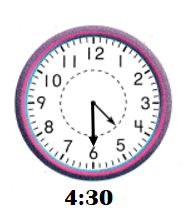# Texas Go Math Grade 1 Lesson 18.4 Answer Key Practice Time to the Hour and Half Hour

Refer to our Texas Go Math Grade 1 Answer Key Pdf to score good marks in the exams. Test yourself by practicing the problems from Texas Go Math Grade 1 Lesson 18.4 Answer Key Practice Time to the Hour and Half Hour.

## Texas Go Math Grade 1 Lesson 18.4 Answer Key Practice Time to the Hour and Half Hour

Explore

Circle the clock that matches the problem.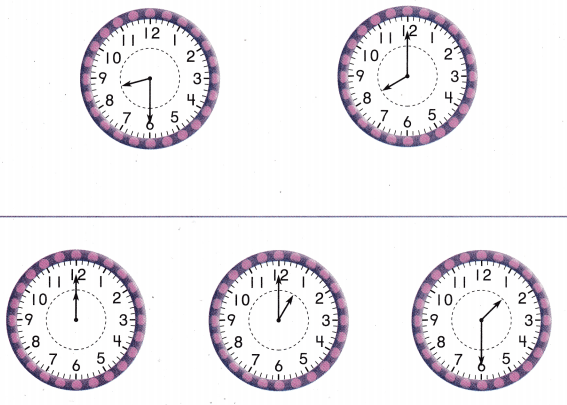FOR THE TEACHER • Read the following problems. Barbara goes to the store at 8:00. Circle the clock that shows 8:00. Children use the top work space to solve. Then have children solve this problem: Barbara takes Ria for a walk at 1:30. Circle the clock shows 1:30.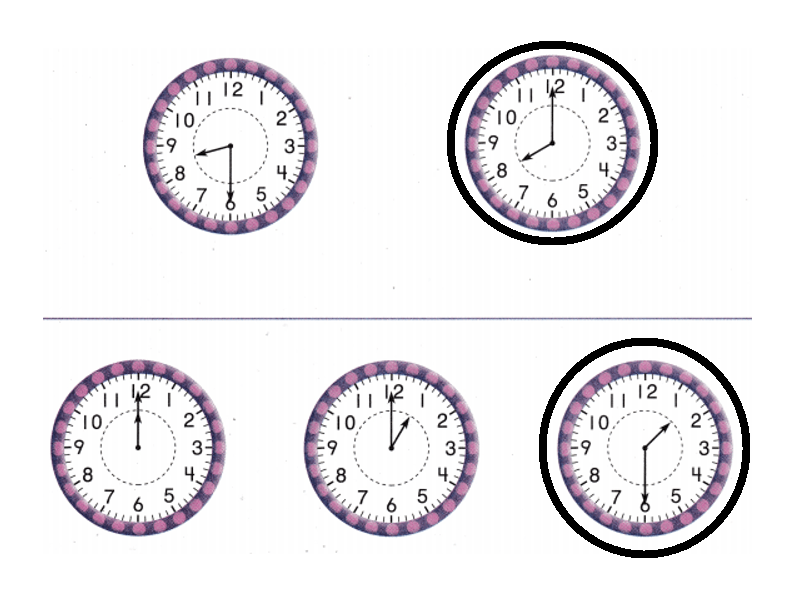Explanation:
Barbara goes to the store at 8:00.
=> Hours hand showing: 8.
Minutes hand showing: 12.
Barbara takes Ria for a walk at 1:30.
=> Hours hand showing: 1.
Minutes hand showing: 6

.

Math Talk
Mathematical Processes
Describe how you know which clock shows 1:30.
Hours hand showing: 1.
Minutes hand showing: 6

Explanation:
Hours hand showing: 1.
Minutes hand showing: 6
By this, clock shows the time 1:30 or half past 1.

Model and Draw
Where should you draw the minute hand to show the time?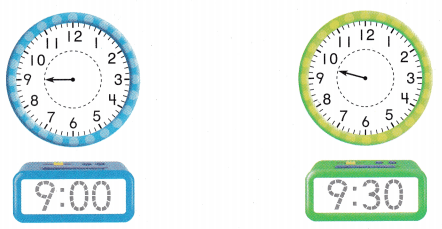Explanation:
Hours hand showing: 9.
Minutes hand showing: 12.
By this, clock shows the time 9:00.
Hours hand showing: 9.
Minutes hand showing: 6.
By this, clock shows the time 9:30 or half past 9.

Share and Show
Use the hour hand to write the time. Draw the minute hand.
Question 1.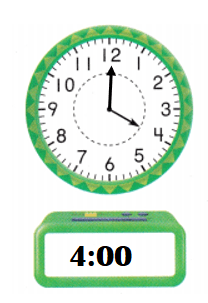Explanation:
Hours hand showing: 4.
Minutes hand showing: 12.
By this, clock shows the time: 4:00.

Question 2.Explanation:
Hours hand showing: 11 and 12 between.
Minutes hand showing: 6.
By this, clock shows the time 11:30 or half past 11.

Question 3.Explanation:
Hours hand showing: 6 and 7 between..
Minutes hand showing: 6.
By this, clock shows the time 6:30 or half past 6.

Question 4.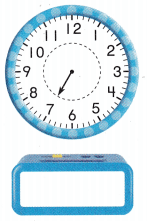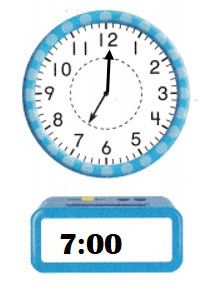Explanation:
Hours hand showing: 7.
Minutes hand showing: 12.
By this, clock shows the time: 7:00.

Question 5.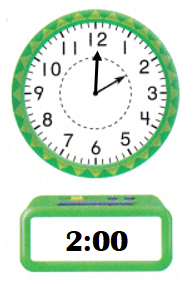Explanation:
Hours hand showing: 2.
Minutes hand showing: 12.
By this, clock shows the time : 2:00.

Question 6.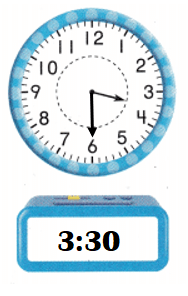Explanation:
Hours hand showing: 3 and 4 between.
Minutes hand showing: 6.
By this, clock shows the time: 3:30 and half past 3.

Problem Solving
Use the hour hand to write the time. Draw the minute hand.
Question 7.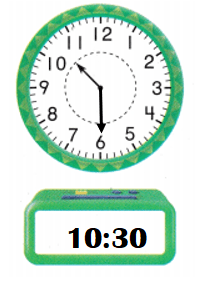Explanation:
Hours hand showing: 10 and 11 between.
Minutes hand showing: 6.
By this, clock shows the time: 10:30 or half past 10.

Question 8.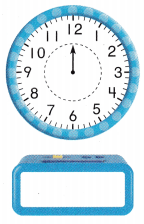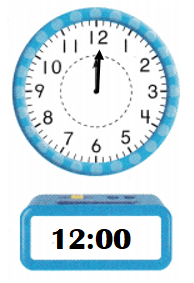Explanation:
Hours hand showing: 12.
Minutes hand showing: 12.
By this, clock shows the time: 12:00.

Question 9.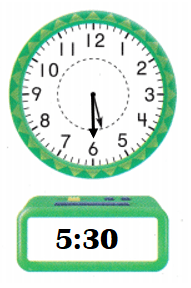Explanation:
Hours hand showing: 5 and 6 between.
Minutes hand showing: 6.
By this, clock shows the time: 5:30 or half past 5.

Question 10.
H.O.T. What is the error? Zoey tried to show 6:00. Explain how to change the clock to show 6:00.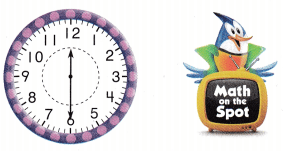Zoey error is that he shown the both hands wrong.

Explanation:
Zoey tried to show 6:00.
Zoey error:
Hours hand showing: 12.
Minutes hand showing: 6.
By this, clock shows the time: 12:30 or half past 12 not 6:00.
Correction:
Hours hand showing: 6.
Minutes hand showing: 12.
By this, clock shows the time: 6:00.

Question 11.
H.O.T. Multi-Step Brandon has lunch at 1 o’clock. Which number does the hour hand point to? Draw the hour hand. Which number does the minute hand point to? Draw the minute hand.Explanation:
Hours hand showing: 1.
Minutes hand showing: 12.
By this, clock shows the time: 1:00.

Question 12.
Sophia and Alana can go on a ride at 4:00. Which clock shows 4:00?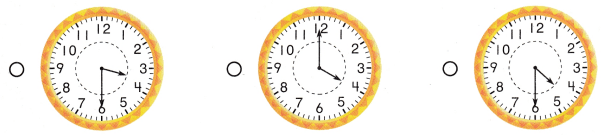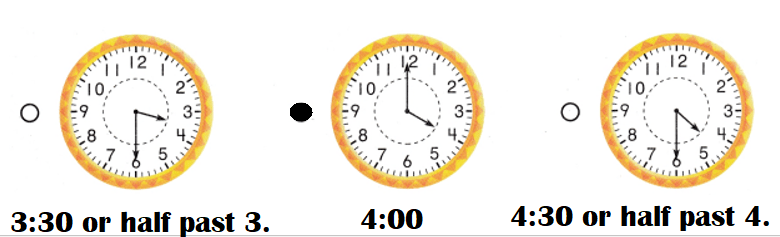Explanation:
Sophia and Alana can go on a ride at 4:00.
Hours hand showing: 4.
Minutes hand showing: 12.
By this, clock shows the time: 4:00.

Question 13.
What time is shown on the clock?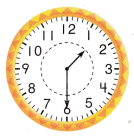(A) 1:00
(B) 12:30
(C) 1:30
Time shown in the clock: 1:30 or half past 1.
(C) 1:30.

Explanation:
Hours hand showing: 1 and 2 between.
Minutes hand showing: 6.
Time shown in the clock: 1:30 or half past 1.

Question 14.
Multi-Step Marybeth draws the clock to show 6:30. What number tells where the minute hand should point? Explain how you know.Explanation:
Marybeth draws the clock to show 6:30.
Hours hand showing: 6 and 7 between.
Minutes hand showing: 6.

Question 15.
Texas Test Prep Which clock shows 11:30?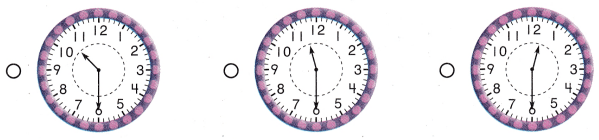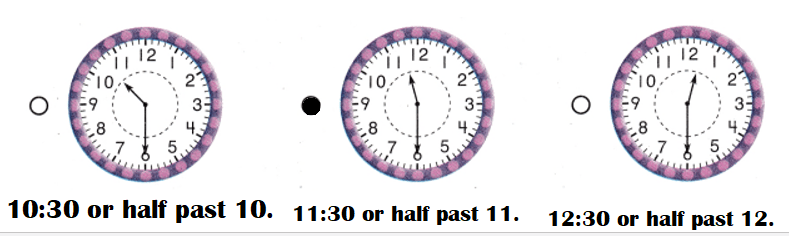Explanation:
Hours hand showing: 10 and 11 between.
Minutes hand showing: 6.
First clock showing the time: 10:30 or half past 10.

Hours hand showing: 11 and 12 between.
Minutes hand showing: 6.
Second clock showing the time: 11:30 or half past 11.

Hours hand showing: 12 and 1 between.
Minutes hand showing: 6.
Third clock showing the time: 12:30 or half past 12.

TAKE HOME ACTIVITY • Show your child the time on the clock to the hour or half hour. Ask him or her to tell you what time is shown.Explanation:
He told:
Hours hand showing: 6 and 7 between.
Minutes hand showing: 6.
Time clock showing: 6:30 or half past 6.

### Texas Go Math Grade 1 Lesson 18.4 Homework and Practice Answer Key

Use the hour hand to write the time. Draw the minute hand.
Question 1.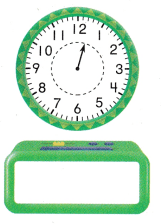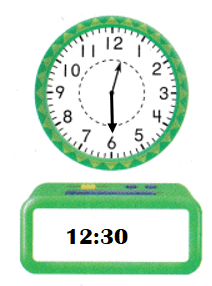Explanation:
Hours hand showing: 12 and 1 between.
Minutes hand showing: 6.
Time clock showing: 12:30 or half past 12.

Question 2.Explanation:
Hours hand showing: 5.
Minutes hand showing: 12.
Time clock showing: 5:00.

Question 3.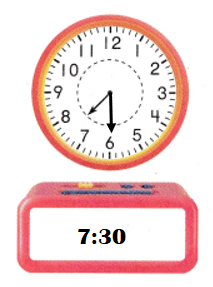Explanation:
Hours hand showing: 7 and 8 between.
Minutes hand showing: 6.
Time clock showing: 7:30 or half past 7.

Problem Solving
Question 4.
What is the error? Russ tried to show 9:30. Explain how to change the clock to show 9:30.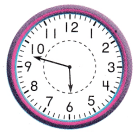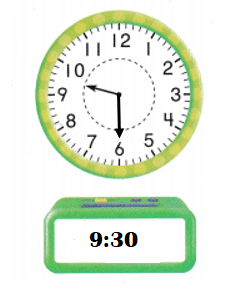Explanation:
Russ tried to show 9:30.
Error:Hours hand and minutes hand both are wrongly shown.
Correction:
Hours hand showing: 9 and 10 between.
Minutes hand showing: 6.
Time clock showing: 9:30 or half past 9.

Lesson Check
Question 5.
Gina and Sean go to the movies at 7:00. Which clock shows 7:00?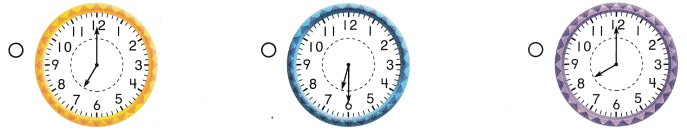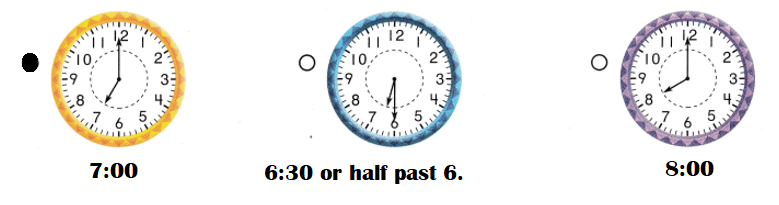Explanation:
Gina and Sean go to the movies at 7:00.
First clock showing time: 7:00.
Second clock showing time: 6:30 or half past 6.
Third clock showing time: 8:00.

Question 6.
What time is shown on the clock?(A) 9:00
(B) 10:30
(C) 9:30
Time shown in the clock : 9:30 or half past 9.
(C) 9:30.

Explanation:
Hours hand showing: 9 and 10 between.
Minutes hand showing: 6.
Time clock showing: 9:30 or half past 9.Question 7.
Which clock shows 2:30?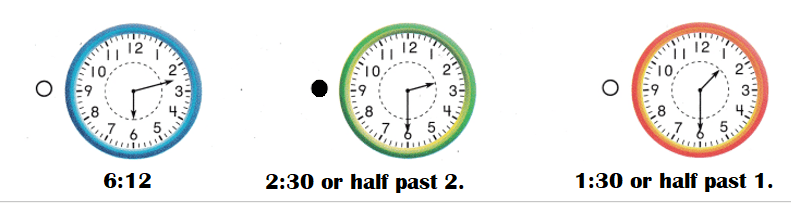Explanation:
First clock showing time: 6:12.
Second clock showing time: 2:30 or half past 2.
Third clock showing time: 1:30 or half past 1.

Question 8.
Multi-Step Lizzy draws the clock to show 4:30. What number tells where the minute hand should point?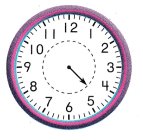(A) 6
(B) 12
(C) 9